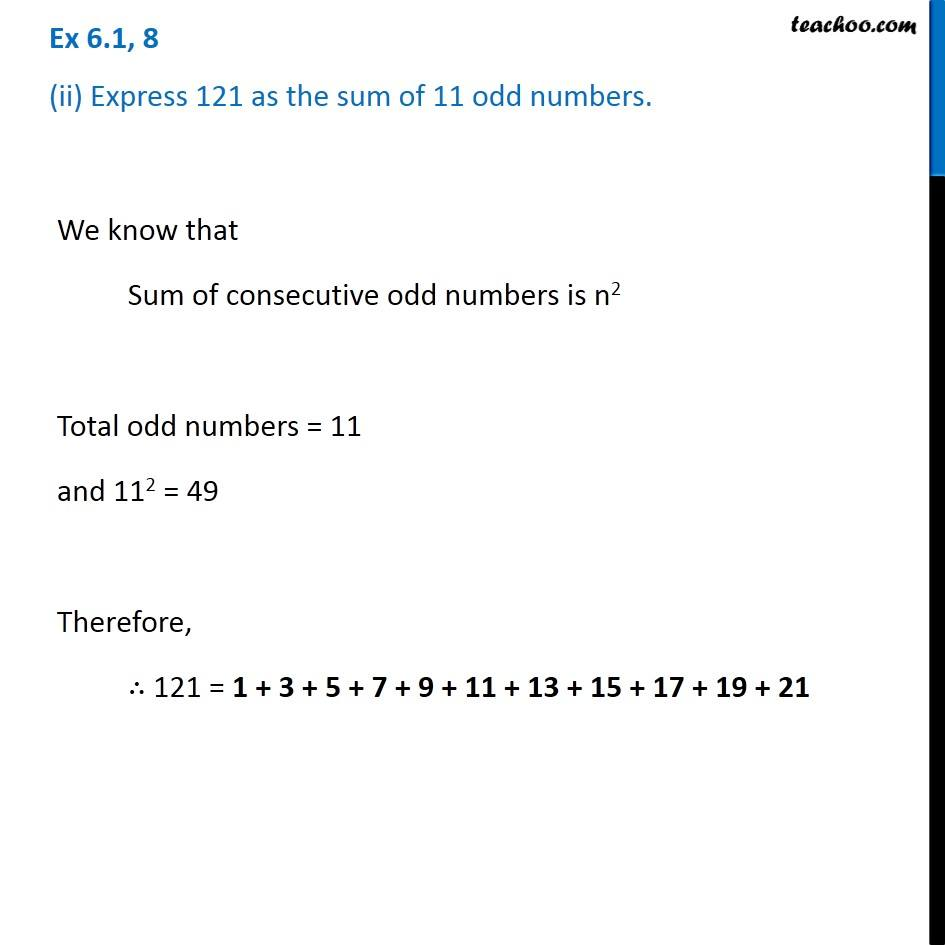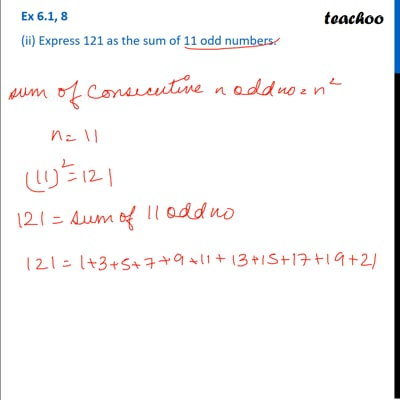Ex 6.1

Chapter 6 Class 8 Squares and Square Roots
Serial order wiseThis video is only available for Teachoo black users

### Transcript

Ex 6.1, 8 (ii) Express 121 as the sum of 11 odd numbers.We know that Sum of consecutive odd numbers is n2 Total odd numbers = 11 and 112 = 49 Therefore, ∴ 121 = 1 + 3 + 5 + 7 + 9 + 11 + 13 + 15 + 17 + 19 + 21